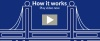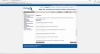Courses - Portage LearningMATH 110: Introduction to Statistics

Credits: 3

\$594.00

Department: Mathematics

A general introduction to mathematical statistics as a tool used in the decision making process. The course is designed to help students develop an understanding of summarized data in both descriptive and inferential statistical applications through the use of frequency distributions, measures of central tendency, measures of dispersion, probability distributions, random sampling, interval estimation, hypothesis testing, comparisons involving means, and regression analysis.

Credits: 3

Prerequisites: Algebra proficiency is required, as demonstrated by successful completion of high school algebra, by completion of a college algebra course, or their equivalent as determined by Portage staff.

Course Topics

Module 1: An introduction to data and statistics. This module discusses why statistics are important, and where statistical analysis is used. Students will learn about different types of data that might be used in statistical analysis. Topics covered include: Quantitative and Qualitative Data, Experimental and Observational Studies, Data Errors, Outliers, Descriptive Statistics, Histograms, Populations, and Samples.

Module 2: An introduction to descriptive statistics using tabular, graphical and numerical methods. This module considers ways to describe and represent data. Topics covered include: Frequency Distributions, Relative Frequencies, Charts (Column, Bar, and Pie), Cross-Tabulation, Scatter Diagrams, Measures of Central Tendency, Percentiles, Quartiles, Measures of Dispersion, Z-scores, Bell Curves, and Sample Covariance.

Module 3: An overview of probability. This module considers experiments and the likelihood that an event will occur. Students are taught to calculate probabilities using multiple techniques. Topics covered include: Probability Distributions, Sample Space, Counting Techniques, Permutations, Combinations, Complements, Union, Intersection, Mutually Exclusive Events, Conditional Probabilities, and Bayes’ Theorem.

Module 4: An introduction to probability distributions. Students will learn about the standard normal probability distribution, and how the data is distributed with respect to the mean. Topics covered include: Random Variables (Discrete and Continuous), Expected Values, Binomial Probability Distributions, Normal Distributions, and The Standard Normal Table.

Module 5: An overview of sampling and sampling distributions. This module explains how to calculate descriptive statistics when working with a sample instead of the entire population. Topics Covered include: Statistical Inference, Simple Random Samples, Sample Mean, Sample Proportions, The Central Limit Theorem, Sample Error, and Sample Size.

Module 6: An introduction to interval estimation. In this module students will learn how to take a sample, find its mean, and use this information to estimate the population mean. Students will be able to construct confidence intervals for the population mean. Topics covered include: Confidence Intervals, Confidence Levels, Means, and Proportions.

Module 7: An introduction to hypothesis testing. In this module students will be guided through the process of hypothesis testing. Students will learn to make assumptions about a certain characteristic of the population and then test to see if the hypothesis is true. Topics covered include: Null Hypothesis, Alternate Hypothesis, One-Tailed and Two-Tailed Tests, Type I and Type 2 errors, and Level of Significance.

Module 8: An introduction to comparisons involving means and proportions. In this module students will study interval estimation and hypothesis testing for differences between two population means as well as for differences between two population proportions. Topics covered include: Dependent Samples, Independent Samples, Hypothesis Testing Involving Differences between Means, and Hypothesis Testing for Dependent Samples.

Module 9: An introduction to regression analysis. Students will learn how to calculate the linear correlation coefficient for a set of data to reveal how well two variables are correlated. Students will also learn how to find the best fit line that approximates the relationship between these variables. Topics covered include: Linear Correlation Coefficients, Positive Correlations, Negative Correlations, Critical Values Correlation Coefficient, and Linear Regression

Module 10: An overview of various tests that were not covered in previous modules. Students will learn about goodness of fit tests, tests for independence, and analysis of variance. Topics covered include: Chi-Square Distributions, F Distributions, Multinomial Experiments, Expected Counts, Goodness of Fit Tests, and Tests for Independence.

Recommended VideosHow it WorksCourse Overview